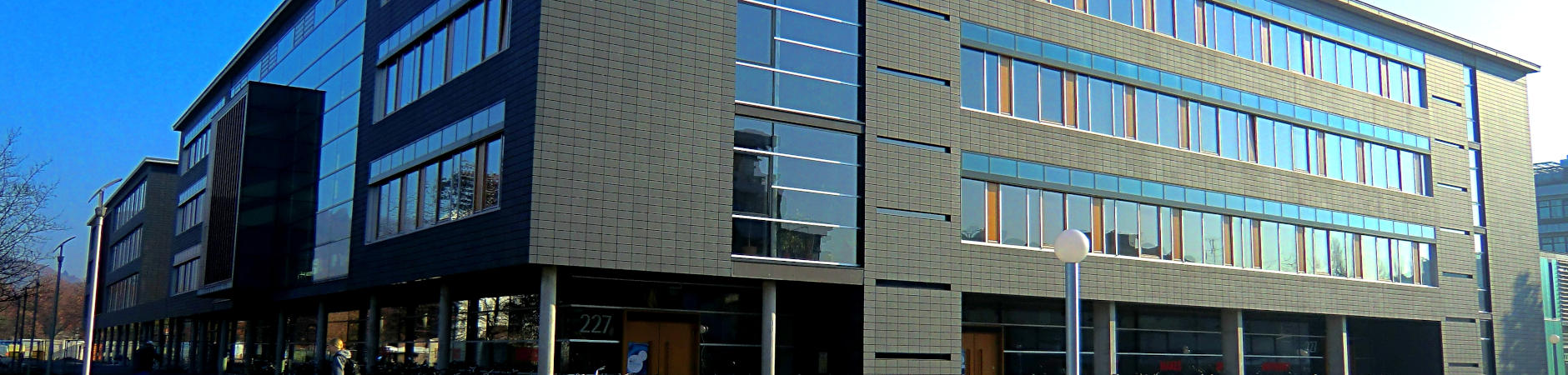# KIP publications

 year 2013 author(s) ATLAS Collaboration title Measurement of dijet cross sections in pp collisions at 7 TeV centre-of-mass energy using the ATLAS detector KIP-Nummer HD-KIP 13-92 KIP-Gruppe(n) F8 document type Paper source arXiv:1312.3524 [hep-ex] Abstract (de) Double-differential dijet cross sections measured in pp collisions at the LHC with a 7 TeV centre-of-mass energy are presented as functions of dijet mass and rapidity separation of the two highest-pT jets. These measurements are obtained using data corresponding to an integrated luminosity of 4.5/fb, recorded by the ATLAS detector in 2011. The data are corrected for detector effects so that cross sections are presented at the particle level. Cross sections are measured up to 5 TeV dijet mass using jets reconstructed with the anti-kt algorithm for values of the jet radius parameter of 0.4 and 0.6. The cross sections are compared with next-to-leading-order perturbative QCD calculations by NLOJET++ corrected to account for non-perturbative effects. Comparisons with POWHEG predictions, using a next-to-leading-order matrix element calculation interfaced to a parton-shower Monte Carlo simulation, are also shown. Electroweak effects are accounted for in both cases. The quantitative comparison of data and theoretical predictions obtained using various parameterizations of the parton distribution functions is performed using a frequentist method. An example setting a lower limit on the compositeness scale for a model of contact interactions is presented, showing that the unfolded results can be used to constrain contributions to dijet production beyond that predicted by the Standard Model. Abstract (en) Double-differential dijet cross sections measured in pp collisions at the LHC with a 7 TeV centre-of-mass energy are presented as functions of dijet mass and rapidity separation of the two highest-pT jets. These measurements are obtained using data corresponding to an integrated luminosity of 4.5/fb, recorded by the ATLAS detector in 2011. The data are corrected for detector effects so that cross sections are presented at the particle level. Cross sections are measured up to 5 TeV dijet mass using jets reconstructed with the anti-kt algorithm for values of the jet radius parameter of 0.4 and 0.6. The cross sections are compared with next-to-leading-order perturbative QCD calculations by NLOJET++ corrected to account for non-perturbative effects. Comparisons with POWHEG predictions, using a next-to-leading-order matrix element calculation interfaced to a parton-shower Monte Carlo simulation, are also shown. Electroweak effects are accounted for in both cases. The quantitative comparison of data and theoretical predictions obtained using various parameterizations of the parton distribution functions is performed using a frequentist method. An example setting a lower limit on the compositeness scale for a model of contact interactions is presented, showing that the unfolded results can be used to constrain contributions to dijet production beyond that predicted by the Standard Model. URL http://arxiv.org/abs/arXiv:1312.3524
Service
KIP - Bibliothek
Im Neuenheimer Feld 227
Raum 3.402
69120 Heidelberg